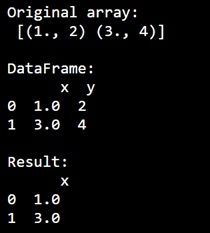# Select records of specific data type from numpy recarray

Learn, how to Select records of specific data type from numpy recarray in Python?
Submitted by Pranit Sharma, on December 31, 2022

NumPy is an abbreviated form of Numerical Python. It is used for different types of scientific operations in python. Numpy is a vast library in python which is used for almost every kind of scientific or mathematical operation. It is itself an array which is a collection of various methods and functions for processing the arrays.

## Selecting records of specific data type from numpy recarray

Suppose we are given a numpy recarray, that has records of different data types or dtypes and we need to select some specific data types from this recarray.

For this purpose, we will first create an array with different data types and then we will create a dataframe using this array. Then dataframe.select_dtypes() method is used to select the specific data types from this numpy recarray.

Let us understand with the help of an example,

## Python code to select records of specific data type from numpy recarray

```# Import numpy
import numpy as np

# Import pandas
import pandas as pd

# Creating a numpy array
arr = np.array([(1.0, 2), (3.0, 4)], dtype=[('x', '<f8'), ('y', '<i8')])

# Display original array
print("Original array:\n",arr,"\n")

# Creating a dataframe
df = pd.DataFrame(arr)

# Display dataframe
print("DataFrame:\n",df,"\n")

# Selecting specific types
res = df.select_dtypes(include=float)

# Display result
print("Result:\n",res,"\n")
```

Output: